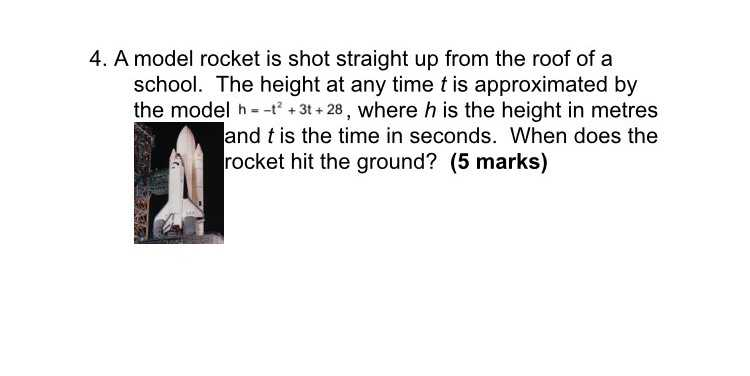### ¿Todavía tienes preguntas de matemáticas?

Pregunte a nuestros tutores expertos
Algebra
Pregunta4. A model rocket is shot straight up from the roof of a school. The height at any time $$t$$ is approximated by the model $$h = - t ^ { 2 } + 3 t + 28$$ , where $$h$$ is the height in metres rocket hit the ground? (5 marks)